# Distillation

## Distillation

### Introduction

Distillation is the most widely-used method of separating fluid mixtures on a commercial scale, it is thus an important part of many processes in the oil and chemical industries.
Many of the tall, thin towers which may be seen in an oil refinery or chemical plant are distillation columns. The most common column diameter is about 2.5 m, but 6 m diameter is commonplace and towers of 12 m dia have been built. Column heights may be as much as 30 m.
The advantages of distillation are: a) High purity products; b) Economies of scale; c) Well-established technology and competitive supply of equipment; d) Use of low temperature, low cost energy; e) Well suited for energy integration into the surrounding process.
It is generally-accepted that if it is possible to achieve a separation by distillation, then distillation will be the most economical method to use. Unless azeotropes are formed (see below), this means that nearly all mixtures where all components have a molecular weight between 140 and 40 will be separated by distillation. Low molecular weight fluids with a critical temperature below 50°C may not be condensed by cheap cooling water, and the additional costs associated with refrigerated or cryogenic distillation may mean that another separation process will be cheaper, at least for small-scale operation. Large molecular weight materials may thermally decompose or polymerize at their boiling temperature even when distilled under a high vacuum. Within the 40 to 140 molecular weight range, distillation is used. In 1992, Darton estimated the world-wide throughput of distillation columns as Oil Refining, 5 billion tonnes per year and petrochemicals, 130 million tonnes per year.
This article describes how distillation columns work, what they contain and how they are designed.

### VAPOR LIQUID EQUILIBRIA

The separation of a mixture by distillation depends on the difference between the compositions of a boiling liquid mixture and the vapor mixture in equilibrium with the liquid. For example, the equilibrium line in Figure 1a correlates the mole fraction of benzene in the vapor, y, in equilibrium with x, the mole fraction of benzene in the liquid for a binary mixture of benzene and toluene. Benzene is more volatile than toluene (i.e., it has a higher vapor pressure at the same temperature) thus at equilibrium, y is greater than x. A distillation column with a boiler at the bottom and a condenser at the top provides a means of countercurrent contact between the rising vapor and the descending liquid, such that at all levels the benzene moves from the liquid into the vapor and the toluene moves from the vapor into the liquid. Thus, benzene concentrates at the top of the column and toluene at the bottom.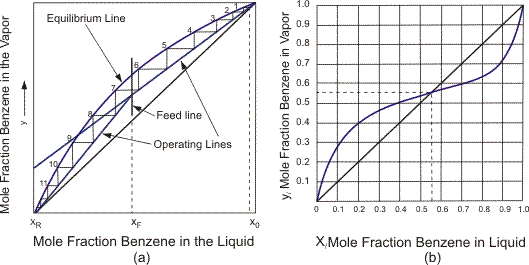Figure 1.
For some mixtures, a constant boiling mixture or azeotrope exists where the composition of the vapor is the same as that of the liquid. For example, the equilibrium line of benzene and ethanol shown in Figure 1b has an azeotrope at 0.55 mol fraction of benzene. This is a low-boiling point azeotrope, i.e., it has a boiling temperature lower than that of both benzene and ethanol. Separation is limited by the composition of the azeotrope.
The prediction of vapor-liquid equilibria ab initio from the molecular structure of the mixture components is not yet possible because a) there is no complete molecular theory of liquids; and b) the equations of state for mixtures of vapors are still essentially empirical. The determination, correlation and prediction of vapor liquid equilibrium have been studied for at least a century and is the subject of specialist texts [Walas (1985) and Reid et al. (1977)]. Collections of experimental data are available [Gmehling and Onken (1977)]. The brief outline of the subject which follows includes the definition of parameters used in designing distillation columns.
For an ideal liquid mixture in contact with a low pressure vapor, the equilibrium compositions may be predicted from Raoult's and Dalton's Law, so that for a component, i,
(1)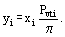For nonideal liquids and higher pressures (approaching but less than the critical pressure), it is necessary to introduce additional terms so that Eq. (1) becomes
(1a)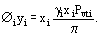The vapor phase fugacity coefficient Øi may be estimated from a suitable equation of state. The liquid phase activity coefficient is more difficult to predict but if some experimental data are available, methods derived from the Gibbs-Duhem Equation are available for predicting or correlating changes in activity coefficients with composition. Activity Coefficients may be predicted from the molecular groups of the components by methods such as the UNIFAC method, now widely-used [Fredenslund et al. (1977)]. A review of methods of predicting vapor liquid equilibrium (V.L.E.) is presented by Prausnitz [Prausnitz (1981)].
The two most commonly-used ways of exploiting V.L.E. in column calcuations are those of the Equilibrium Constant or K-value, and relative volatility, a. The K-value provides a linear relationship for each component between its concentrations in the vapor and liquid, that is y = Kx. Thus from Eq. (1) or (1a)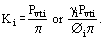A component's K-value thus varies with both temperature and pressure. In general, either temperature or pressure is specified. The procedure for calculating the composition of, say, the vapor in equilibrium with a known composition multicomponent liquid is based on adjusting (by trial) the other variable, such that ∑yi = 1.0 where yi = Kixi. In distillation column calculations, the variable is usually temperature so that the equilibria calculations yield a column temperature profile. The complex nature of this calculation means that nowadays all multicomponent column designs are done by means of computer-based numerical methods [see, for example, Prausnitz et al. (1980)].
Relative volatility is defined as the ratio of the K-value of one component to that of another, that is: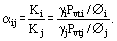For ideal mixtures, αij = Pvti/Pvtj. Both vapor pressures Pvti and Pvtj depend strongly on temperature, but their ratio, αij, is often relatively constant throughout the column. Relative volatility provides an estimate of the difficulty of a particular separation, i.e., α = 10 for easy separation, α = 1.1 for difficult separation.
The dependence of vapor pressures on temperature provides the link between the difficulty of a particular separation and the difference in normal boiling points of the components, i.e., 20°C difference for easy separation; 1°C difference, difficult separation. A difficult separation requires a large column and a large energy input.

### Design of Distillation Columns

The procedure is introduced by first considering a binary mixture; Figure 2 shows a distillation column. Some of the liquid from the condenser at the top of the column, Lc, is returned as reflux. The reflux ratio is defined as the ratio of the liquid returned to the column divided by the liquid removed as product, i.e., R = Lc/D. Figure 2 also shows the column as a series of theoretical plates. A theoretical plate is defined as a vapor-liquid contacting device such that the vapor leaves it in equilibrium with the liquid which leaves it. The first stage of column design is to calculate a) the column reflux ratio; and b) the number of theoretical plates.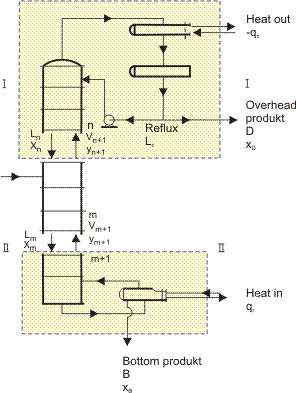Figure 2.
The theoretical plates in Figure 2 are numbered from the top of the column, and streams leaving a plate (in equilibrium) have the number of that plate. Thus, stream V2 leaving plate 2 has vapor concentration y2 and L2 has liquid concentration x2. If y2 and x2 refer to benzene in the benzene-toluene binary mixture, then y2 and x2 are each located at the same point on the equilibrium curve of Figure 1a.
A mass balance may be used to obtain a relationship between the concentrations of any component in the streams passing each other countercurrently between the plates and the product streams leaving the ends of the column. Thus for the column section above the feed in Figure 2, between plates 1 and 2,
(2)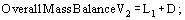(2a)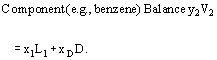In general, a heat balance is also required to allow for changes in L and V as the composition changes, but in most cases, it is reasonable to assume that the molar latent heats of all components of the mixture are approximately equal and that as much liquid evaporates as vapor condenses. That is, L and V are assumed to be constant between the top of the column and the feed, and constant (but different) between the feed and the bottom of the column. Thus for the section above the feed, the mass balances becomes: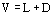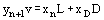(3)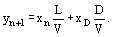Below the feed, in a similar way, the following equations may be derived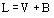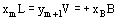(4)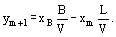Note that for all sections of a particular column that (xDD/V) and (xB B/V) remain constant, the component mass balance Equations (3) and (4) maybe drawn as straight lines (Operating Lines) below the Equilibria Line (Figure 1). Each has a slope equal to its' (L/V).
The flow rate ratios are related to the reflux ratio so that Equation (3) may be written as:
(5)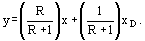In general, the calculation of the number of theoretical plates required for a given separation at a given reflux ratio proceeds as follows: from a known vapor composition leaving a plate (say plate 1 where y1 = xD), use the theoretical plate concept and V.L.E. data to calculate the composition of the liquid leaving the plate (say x1); then use a mass balance [say Equation (3)] to calculate the composition of the vapor leaving the plate below (y2 from plate 2). Repeat the calculation using theoretical plate-mass balance to get the composition of vapor leaving the next plate (say y3 from plate 3) until the composition matches that of the feed. Then adjust the mass balance equations and deal with the section of the column below the feed.
These same principles are applied for multicomponent mixtures, and for changes in flow rates caused by taking off product at intermediate positions (e.g., sidestreams) or multiple feeds. They are well-described in many text books [Coulson and Richardson (1978) and Treybal (1981)]. The case of a binary mixture may be used to explain the meaning of Total Reflux and Minimum Reflux Ratio. Note that for a binary mixture, the process may be represented graphically as in Figure 1 and the number of theoretical plates obtained by stepping off plates between the Operating Lines and the Equilibrium Line (known as the McCabe–Thiele method).
Total Reflux is the operating condition where vapor and liquid are passing each other in the column but no product is removed (i.e., D = 0 and R = L/D = ∞ ). The slopes of the Operating Lines are then L/V = 1.0, that is by mass balance, between each plate y = x. At total reflux, the number of theoretical plates required is a minimum. As the reflux ratio is reduced (by taking off product), the number of plates required increases. The Minimum Reflux Ratio (R min) is the lowest value of reflux at which separation can be achieved even with an infinite number of plates. It is possible to achieve a separation at any reflux ratio above the minimum reflux ratio. As the reflux ratio increases, the number of theoretical plates required decreases.
The Optimum Reflux Ratio (R0) is that at which the total cost of the distillation is a minimum, taking into account the capital cost of the column (which depends on the number of theoretical plates) and running cost, which depends on the reflux ratio. Note that the capital costs of the reboiler and condenser also depend on the reflux ratio. Thus, usually for very low energy costs R0/Rmin = 1.3, and for high energy cost R0/Rmin = 1.1 (it is best not to use R0/Rmin < 1.1 to allow for possible errors in the V.L.E data).

### Column Internals, Trays and Packings

Examples of trays and packings are shown in Figures 3a and b. Trays (or plates) consist of horizontal plates across which the descending liquid flows through streams of the ascending vapor, which is distributed across the column by various sorts of perforations (holes, valves, bubblecaps) in the plates.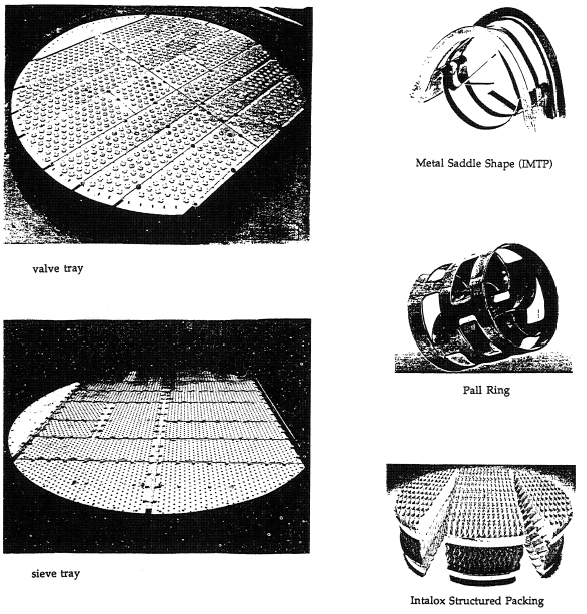Figure 3.
Packings provide surfaces over which the liquid flows while the vapor passes between these surfaces.
The number of real trays required (N) is related to the number of theoretical trays (NTP) by the concept of tray efficiency, E0 = (NTP)/N.
The height of the column occupied by trays is then Z = N. (T.S.) where (TS) is the tray spacing, which is usually 300 mm, 450 mm, or 600 nun except in cryogenic distillation where (TS) is 100 to 150 mm.
The height of the column containing packing is usually calculated by Z = (NTP) (HETP), where (HETP) = Height of Packing Equivalent to One Theoretical Plate.

### Column Diameter Pressure Drop and Flooding

To keep the column diameter (and cost) as small as possible, columns are designed to operate at the maximum permissible vapor velocity. Except for those cases where pressure drop is controlling [see Strigle R.F. (1994)], this is usually at about 80% of the flooding velocity. (See Flooding and Flow Reversal.) At flooding vapor velocity, the pressure gradients and drag forces overcome the gravitational forces on the liquid and it ceases to descend the column. Flooding may be correlated in terms of the Load Factor (CS) and the Flow Parameter (X) [see Figures 4a and 4b], where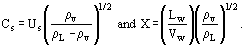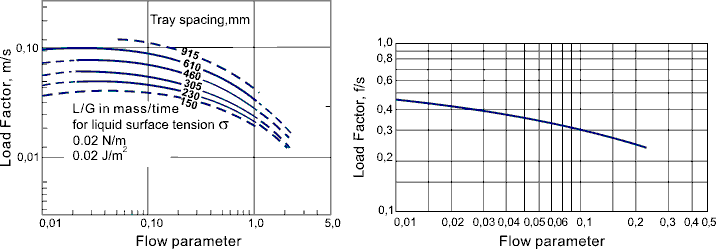Figure 4.
The Load Factor CS is very approximately proportional to G/(√ρv) and this, in part, explains the important practical observation that throughput—expressed as a mass flow per unit column cross section area—goes up with pressure. The mass of liquid descending is similar to that of vapor ascending. Typical values of liquid flow per unit area are given in the following table.

### Tray Efficiency and HETP

A great deal has been written on tray and packing, mass transfer in trays and packings. Tray mass transfer is interpreted in terms of a) Point Efficiency, Eog; b) Murphree Tray Efficiency, Emv; and c) Overall Column Efficiency, Eo. Point efficiency refers to mass transfer at a point on the tray and is related to single-tray or Murphree Efficiency, taking into account the flow pattern on the tray. Overall column efficiency is then obtained by considering concentration (and flow patterns) throughout the column. Reference can be made to Lockett (1986). Packing mass transfer is interpreted in terms of vapor and liquid mass transfer coefficients, and sometimes by the transfer unit concept [see, for example, Kister (1989) and Strigle (1994)]. Here, only general comments can be made. Tray Efficiency does not change much with the type of tray or tray spacing, but varies with operating pressure being lower for vacuum distillation than for pressure distillation. This reflects the changes in liquid rate mentioned above (0.5 bar, Eo approx 0.5; 1.0 bar, Eo approx 0.7; 6 bar, Eo approx 0.9).
Packing HETP might be expected to go down with pressure but, in practice, does not change much with a system for a given packing. However, it changes with packing size, which determines the dry area per unit volume. For example, for random packings, HETP (m) approximately equals dp/60 (where dp is packing size in mm). A high quality of the initial distribution of liquid and vapor is essential in large-diameter columns (even in those larger than 0.5 m). Without this scale up, failures have occurred. In general, a column with more theoretical trays for a given height will require a larger diameter, that is closer tray spacings or high area packings flood at a lower throughput. The design of distillation columns is still essentially empirical, in part based on avoiding earlier failures. (For example, there is no theoretical basis so far for two-phase flow on trays or in packings, nor is there a theory for multicomponent mass transfer on cross-flow trays. )
Theory and practice are interpreted by Porter and Jenkins in a paper which explains why it is relatively easy to correlate distillation experience even in terms of the wrong theory.

### Process Design (Sequencing Distillation Columns)

A simple continuous distillation column separates a feed into two products (top product and bottom product); thus, complete separation in one column is possible only for a binary mixture. For a three-component mixture (say ABC), a complete separation may be achieved in either one of two alternative sequences each of two columns, (that is A/BC, B/C or AB/C, A/B). The process design problem is to choose which of these sequences is the optimum from the point of view of cost or of suitability for energy integration. For a mixture of n components, the number of columns required in each alternate sequence is always (n–1), but the number of possible sequences goes up rapidly with the number of components, e.g., n = 3, S = 2; n = 4, S = 5; n = 6, S = 42, n = 10, S = 3,500). Choosing the optimum sequence is thus a complex problem which has received much attention. It is solved either by sophisticated numerical procedures to minimize the number of complete design and costings to be performed, or by oversimplifying rules of thumb which sometimes contradict each other. The best known rules are: a) Do the easiest separation first and the hardest last; b) separate in order of volatilities (the direct sequence); c) favor 50/50 splits: and d) remove the most plentiful component first. A simple equation proposed by Porter and Momoh has been found to identify the best sequence for energy integration and an example of its use is given by Porter, Jenkins and Momoh (1987).

### Nomenclature

B Molar flow of bottom product (kg mol/h)
CS Vapor Load Factor = us (ρv/(ρL − ρv))1/2 (m/s)
D Molar Flow of top product (distillate) from the condenser (kg mol/h)
Eog Point Efficiency on a Tray
Emv Single Tray Murphree Efficiency
Eo Overall column tray efficiency
G Mass flow of vapor per unit column cross-sectional area (kg/s·m2)
HETP Height of Packing Equivalent to a Theoretical Plate (m)
K Equilibrium Ratio (y/x)
L Molar liquid flow in a section of the column (kg mol/h)
Lc Molar liquid flow to the top of the column (kg mol/h)
Lw Mass liquid flow in a section of the column (kg/h)
N Number of real trays (or plates) in a distillation column
n Number of components in the mixture
Pvti Vapor pressure of component i at temperature t (bar)
R Reflux ratio (Lc/D)
Rmin Minimum Reflux Ratio
Ro Optimum Reflux Ratio
S Number of possible sequences to separate a multicomponent mixture
TS Tray Spacing (m)
us Superficial (i.e., empty tower) vapor velocity (m/s)
V Molar vapor flow in a section of the column (kg·mol/h)
Vw Mass vapor flow in a section of the column (kg/h)
V.L.E. Vapor Liquid Equilibria (refers to vapor and liquid concentrations)
x Mol. fraction of a component in the liquid
y Mol. fraction of a component in the vapor
Z Height of the column which contains trays or packing (m)
αij Relative volatility of component i to that of j
γ Activity coefficient in the liquid
Ø Fugacity coefficient in the vapor
π Total pressure of the system (bar)
ρv, ρi Densities of vapor, liquid kg/m3
i, j Subscripts refering to components i, j, etc.